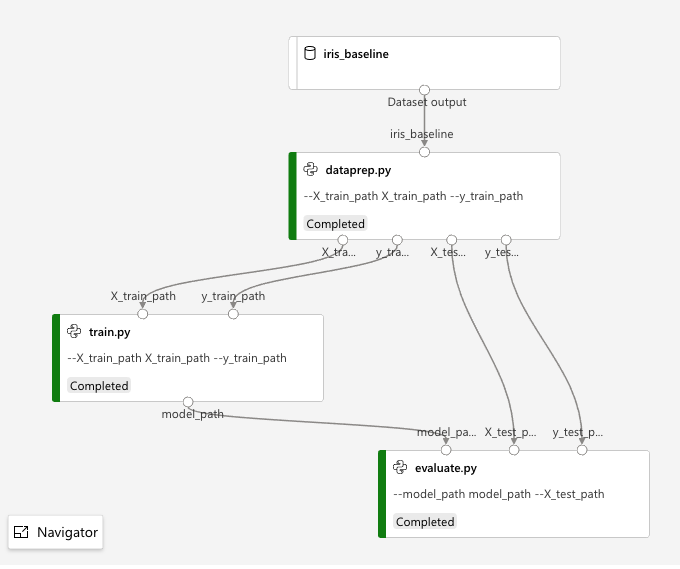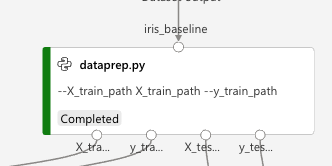# A Simple 3-Step AzureML Pipeline (Dataprep, Training, and Evaluation)

Get the source code and data on GithubThis demonstrates how you create a multistep AzureML pipeline using a series of `PythonScriptStep` objects.

In this case, the calculation is extremely trivial: predicting Iris species using scikit-learn's Gaussian Naive Bayes. This pipeline could be solved (very quickly) using this code:

```import pandas as pd
from sklearn.naive_bayes import GaussianNB
from sklearn.model_selection import train_test_split
from sklearn.metrics import accuracy_score

# These two lines become the data ingestion and dataprep steps
X_train, X_test, y_train, y_test = train_test_split(df.iloc[:,1:4], df.iloc[:,4:5], test_size=0.2, random_state=42)

# These two lines become the training step
model = GaussianNB()
model.fit(X_train, y_train.values.ravel())

# These two lines become the evaluation step
prediction = model.predict(X_test)
print(f'Accuracy: {accuracy_score(prediction, y_test):3f}')
```

The point of this notebook is to show the construction of the AzureML pipeline, not demonstrate any kind of complex machine learning.

## Preliminary setup

Import types used:

```from azureml.core import Environment, Experiment, Workspace, Datastore, Dataset
from azureml.core.compute import ComputeTarget, AmlCompute
from azureml.core.runconfig import RunConfiguration
from azureml.data import OutputFileDatasetConfig
from azureml.data.datapath import DataPath
from azureml.pipeline.core import Pipeline
from azureml.pipeline.steps import PythonScriptStep

from sklearn.metrics import accuracy_score
from sklearn.model_selection import train_test_split
from sklearn.naive_bayes import GaussianNB
from sklearn.preprocessing import LabelEncoder

import pandas as pd
```

This notebook requires `azureml.core.VERSION >= 1.12.0`

```import azureml.core
azureml.core.VERSION
```
```'1.12.0'
```
• Access the AzureML workspace (relies on `config.json` downloaded from workspace in same dir as notebook).

• Retrieve the default datastore for the workspace. This is where the `Dataset` (permanent data) and temporary data will be stored.

```ws = Workspace.from_config()
ds = ws.get_default_datastore()
```

# Data Ingestion

Register the data as a `Dataset` within the ML workspace, if necessary. Relies, initially, on the presence of the iris dataset in the local `./data` dir.

```baseline_dataset_name = 'iris_baseline'

if not baseline_dataset_name in Dataset.get_all(ws).keys() :
iris_dataset = Dataset.Tabular.from_delimited_files(DataPath(ds, 'iris_data_baseline/iris.csv'))
iris_dataset.register(ws, 'iris_baseline', description='Iris baseline data (w. header)')
```

## Compute Resource & Python Environment

For this super-easy problem, just use a CPU-based cluster and share the environment between pipeline steps. The curated environment `AzureML-Tutorial` happens to have `sklearn`, so that's why I chose it.

```compute_name = "cpu-cluster2"
vm_size = "STANDARD_D2_V2"
if compute_name in ws.compute_targets:
compute_target = ws.compute_targets[compute_name]
if compute_target and type(compute_target) is AmlCompute:
print('Found compute target: ' + compute_name)
else:
print('Creating a new compute target...')
provisioning_config = AmlCompute.provisioning_configuration(vm_size=vm_size,  # STANDARD_NC6 is GPU-enabled
min_nodes=0,
max_nodes=4)
# create the compute target
compute_target = ComputeTarget.create(
ws, compute_name, provisioning_config)
```
```Found compute target: cpu-cluster2
```
```env = Environment.get(ws, "AzureML-Tutorial")

runconfig = RunConfiguration()
runconfig.target = compute_target
runconfig.environment = env
```

## Data preparation and augmentation step

Now that the `Dataset` is registered, it's available for use.

```iris_dataset = Dataset.get_by_name(ws, 'iris_baseline')
iris_dataset.take(3).to_pandas_dataframe()
```
sepal_length sepal_width petal_length petal_width species
0 5.1 3.5 1.4 0.2 Iris-setosa
1 4.9 3.0 1.4 0.2 Iris-setosa
2 4.7 3.2 1.3 0.2 Iris-setosa
```ds_input = iris_dataset.as_named_input("iris_baseline")
```
• Use `Dataset`s for initial input to a Pipeline.
• Use `OutputFileDatasetConfig` for temporary data that flows between pipeline steps.
```X_train_dir = OutputFileDatasetConfig("X_train_dir")
X_test_dir = OutputFileDatasetConfig("X_test_dir")
y_train_dir = OutputFileDatasetConfig("y_train_dir")
y_test_dir = OutputFileDatasetConfig("y_test_dir")
```

Create the dataprep step:Note how the `Dataset` input and `OutputFileDatasetConfig` outputs are shown.

```# I set reuse to `False`, since part of this step is random selection of sets. ('Cept, of course, RANDOM_SEED is the same)
dataprep_step = PythonScriptStep(
script_name = "dataprep.py",
arguments=[
"--X_train_dir", X_train_dir,
"--y_train_dir", y_train_dir,
"--X_test_dir", X_test_dir,
"--y_test_dir", y_test_dir],
inputs = [ds_input],
compute_target = compute_target,
source_directory="./src/dataprep",
allow_reuse = False,
runconfig = runconfig
)
```

## Training

The next step takes two outputs from the first step and writes the model to the `model_path` output.

```model_dir = OutputFileDatasetConfig("model_path")

training_step = PythonScriptStep(
script_name = "train.py",
arguments=[
"--X_train_dir", X_train_dir.as_input("X_train_dir"),
"--y_train_dir", y_train_dir.as_input("y_train_dir"),
"--model_dir", model_dir],
compute_target = compute_target,
source_directory="./src/train/",
allow_reuse = True,
runconfig=runconfig
)
```

## Evaluation

Takes the `model_path` from the training step and the test data from the dataprep step. Internally, it reconstitutes the model, runs it against the test data, and writes something to the log (the child run's `70_driver_log.txt` file).

```eval_step = PythonScriptStep(
script_name = "evaluate.py",
arguments=[
"--model_dir", model_dir.as_input("model_dir"),
"--X_test_dir", X_test_dir.as_input("X_test_dir"),
"--y_test_dir", y_test_dir.as_input("y_test_dir")],
compute_target = compute_target,
source_directory="./src/evaluate/",
allow_reuse = True,
runconfig=runconfig
)
```

## Create pipeline

• The source code associated with the individual steps is zipped up, uploaded.
• The compute resource is allocated
• A Docker image is built for it w. the necessary environment
• The dependency graph for the pipeline is calculated
• The steps execute, as necessary

In this case, since I set `allow_reuse` to `False` in the first step, every run will cause a total rerun. The thing is that my very first step is where I do not just datapreparation, but the shuffling for the test/train split. That could be split into multiple steps if dataprep were an expensive operation. Or, if datapreparation manipulated both testing and training data, then you could have dataprep be one step and do the test/training split either at the beginning of the train step or as a separate step.

I could imagine for instance, after the test/train split, you put the same data into two different training steps, which you directly compare in the evaluation split...

But all of that goes beyond this simple example...

```# Build the pipeline
pipeline1 = Pipeline(workspace=ws, steps=[dataprep_step, training_step, eval_step])
# Submit the pipeline to be run
pipeline_run1 = Experiment(ws, 'Iris_SKLearn_Pipeline').submit(pipeline1)
pipeline_run1.wait_for_completion()
```
```Created step dataprep.py [1c75fd38][e8dd26b8-a147-4934-9a4f-42bbe563a84d], (This step will run and generate new outputs)
```

...etc...

### Last posts

1. Longer Orange 30 SLA Printer Assembly & Initial Impressions
2. From Whalesharks To Leopard Spheres

tags: MLSD Futures

3. Humpback Whale Identification By Deep Learning
4. Please: Say "Twin" Networks, Not "Siamese" Networks

tags: ML

5. Ricky Jay R.I.P